# 1 Introduction

This vignette is intended to help users to start using the DRomics package. It is complementary to the reference manual where you can find more details on each function of the package. The first part of this vignette (Main workflow, steps 1 to 4) could also help users of the Shiny application. If you do not want to use R functions, you can skip the pieces of code and focus on the explanations and on the outputs that are also given in the Shiny application. And if one day you want go further using the R functions, we recommend you to start from the whole R code corresponding to your analysis that is provided on the last page of the Shiny application.

# 2 Main workflow

## 2.1 Step 1: importation, check and normalization / transformation of data if needed

### 2.1.1 General format of imported data

Whatever the type of data imported in DRomics, data can be imported from a .txt file (e.g. “mydata.txt”) containing one row per item, after the first row coding for doses or concentrations for each sample (each column except the first one), with the first column corresponding to the identifier of each item (identifier of the probe, transcript, metabolite, …, or name of the endpoint for anchoring data), and the other columns giving the responses of the item for each sample. In the first row, after a name for the identifier column, we must have the tested doses or concentrations in a numeric format for the corresponding sample (for example, if there are triplicates for each treatment, the first line could be “item”, 0, 0, 0, 0.1, 0.1, 0.1, etc.). This file is imported within DRomics using the function read.table() with its default field separator (sep argument).

Alternatively an R object of class data.frame can be directly given in input, corresponding to the output of read.table(file, header = FALSE) on a file described as above.

You can see below an example of a RNAseq data set that is available in DRomics both as an R object (named Zhou_kidney_pce) and as a text file named “RNAseq_sample.txt” containing just a sample of the previous one.

``````# Load and look at the first line of the R object
data(Zhou_kidney_pce)
nrow(Zhou_kidney_pce)``````
``##  33395``
``head(Zhou_kidney_pce)``
``````##             V1   V2   V3      V4      V5      V6      V7      V8      V9  V10
## 1       RefSeq    0    0    0.22    0.22    0.22    0.67    0.67    0.67    2
## 2    NM_144958 2072 2506 2519.00 2116.00 1999.00 2113.00 2219.00 2322.00 2359
## 3    NR_102758    0    0    0.00    0.00    0.00    0.00    0.00    0.00    0
## 4    NM_172405  198  265  250.00  245.00  212.00  206.00  227.00  246.00  265
## 5    NM_029777   18   29   25.00   19.00   19.00   13.00   22.00   19.00   19
## 6 NM_001130188    0    0    0.00    0.00    0.00    0.00    0.00    1.00    0
##    V11  V12  V13  V14  V15
## 1    2    2    6    6    6
## 2 1932 1705 2110 2311 2140
## 3    0    0    0    0    0
## 4  205  175  288  315  242
## 5   26   16   26   32   33
## 6    0    0    1    0    1``````
``````# Import the text file just to see what will be automatically imported
datafilename <- system.file("extdata", "RNAseq_sample.txt", package = "DRomics")
# for your local file datafilename choose the name: "yourchosenname.txt"
nrow(d)``````
``##  1000``
``head(d)``
``````##             V1   V2   V3      V4      V5      V6      V7      V8      V9  V10
## 1       RefSeq    0    0    0.22    0.22    0.22    0.67    0.67    0.67    2
## 2    NM_144958 2072 2506 2519.00 2116.00 1999.00 2113.00 2219.00 2322.00 2359
## 3    NR_102758    0    0    0.00    0.00    0.00    0.00    0.00    0.00    0
## 4    NM_172405  198  265  250.00  245.00  212.00  206.00  227.00  246.00  265
## 5    NM_029777   18   29   25.00   19.00   19.00   13.00   22.00   19.00   19
## 6 NM_001130188    0    0    0.00    0.00    0.00    0.00    0.00    1.00    0
##    V11  V12  V13  V14  V15
## 1    2    2    6    6    6
## 2 1932 1705 2110 2311 2140
## 3    0    0    0    0    0
## 4  205  175  288  315  242
## 5   26   16   26   32   33
## 6    0    0    1    0    1``````

### 2.1.2 Types of data that may be imported in DRomics

DRomics offers the possibility to work on different types of omics data (see next paragraph for their description) but also on continuous anchoring data. When working on omics data, all the lines of the dataframe (except the first one coding for the doses or concentrations) correspond the same type of data (e.g. raw counts for RNAseq data). When working on anchoring data, the different lines (except the first one coding for the doses or concentrations) correspond to different endpoints that may correspond to different types of data (e.g. biomass, length,..), but all are assumed continuous data compatible with a normal error distribution (e.g. after logarithmic transformation for metabolomic data) for the selection and modelling steps.

Three types of omics data may be imported in DRomics using the following functions:

• RNAseqdata() should be used to import RNAseq as counts of reads,
• microarraydata() should be used to import single-channel microarray data in log2 scale,
• continuousomicdata() should be used to import other continuous omics data such as metabolomics, proteomics,…, in a scale that enables the use of a normal error model in Steps 2 and 3. metabolomicdata() is the former name, but still available, of this function.

In Steps 1 and 2 count data are internally analysed using functions of the Bioconductor package DESeq2 while continuous data (microarray data and other continuous omics data) are internally analysed using functions of the Bioconductor package limma.

### 2.1.3 An example with RNAseq data

``RNAseqfilename <- system.file("extdata", "RNAseq_sample.txt", package = "DRomics")``

For RNAseq data, imperatively imported in raw counts, you have to choose the transformation method used to stabilize the variance (“rlog” or “vst”). In the example below “vst” was used only to make this vignette quick to compile, but “rlog” is strongly recommended and chosen by default even if more computer intensive than “vst” (see ?RNAseqdata for details). Whatever the chosen method, data are automatically normalized with respect to library size and transformed in a log2 scale.

``(o.RNAseq <- RNAseqdata(RNAseqfilename, transfo.method = "vst"))``
``````## Elements of the experimental design in order to check the coding of the data:
## Tested doses and number of replicates for each dose:
##
##    0 0.22 0.67    2    6
##    2    3    3    3    3
## Number of items: 999
## Identifiers of the first 20 items:
##   "NM_144958"    "NR_102758"    "NM_172405"    "NM_029777"    "NM_001130188"
##   "NM_207141"    "NM_001162368" "NM_008117"    "NM_001168290" "NM_010910"
##  "NM_001004147" "NM_001146318" "NM_145597"    "NM_001161797" "NM_021483"
##  "NR_002862"    "NR_033520"    "NM_134027"    "NM_010381"    "NM_019388"
## Data were normalized with respect to library size and tranformed using
## the following method: vst``````
``plot(o.RNAseq, cex.main = 0.8, col = "green", range = 1e6)``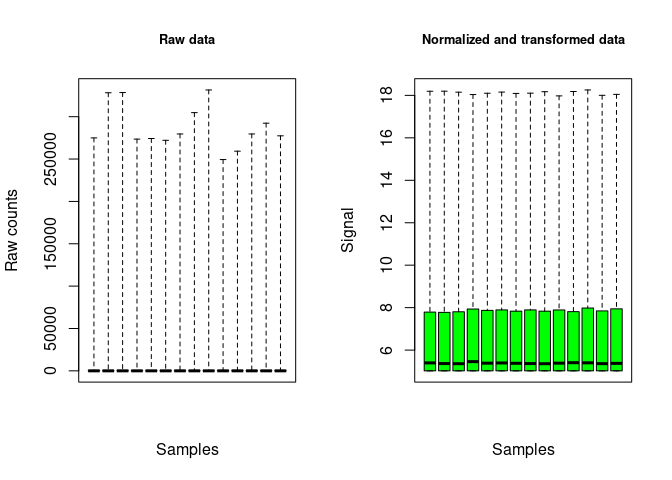In the previous example the argument range (internally passed to boxplot) is put to a high value just to plot true minimum and maximum values and to prevent the automatic plot of many outliers as individual points in the plot of raw counts.

### 2.1.4 An example with microarray data

For single-channel microarray data, imperatively imported in log scale (classical and recommended log2 scale), you can choose the between array normalization method (“cyclicloess”, “quantile”, “scale” or “none”). In the example below “quantile” was used only to make this vignette quick to compile, but “cyclicloess” is strongly recommended and chosen by default even if more computer intensive than the others (see ?microarraydata for details).

``microarrayfilename <- system.file("extdata", "transcripto_sample.txt", package = "DRomics")``
``(o.microarray <- microarraydata(microarrayfilename, norm.method = "quantile"))``
``````## Elements of the experimental design in order to check the coding of the data:
## Tested doses and number of replicates for each dose:
##
##     0  0.69 1.223 2.148 3.774 6.631
##     5     5     5     5     5     5
## Number of items: 1000
## Identifiers of the first 20 items:
##   "1"    "2"    "3"    "4"    "5.1"  "5.2"  "6.1"  "6.2"  "7.1"  "7.2"
##  "8.1"  "8.2"  "9.1"  "9.2"  "10.1" "10.2" "11.1" "11.2" "12.1" "12.2"
## Data were normalized between arrays using the following method: quantile``````
``plot(o.microarray, cex.main = 0.8, col = "green", range = 1e6)``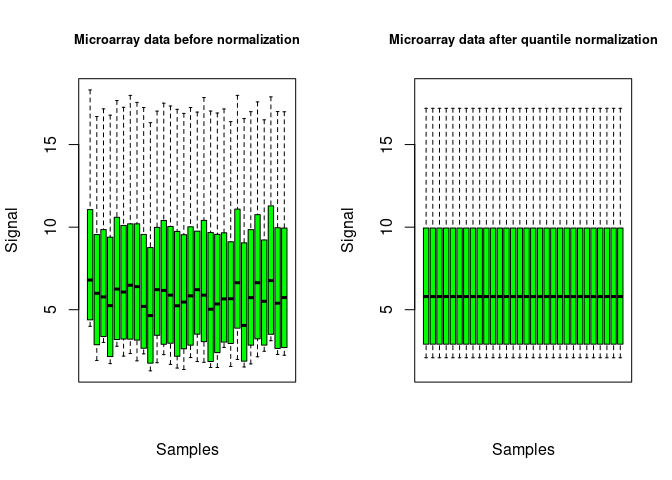In the previous example the argument range is intended to be internally passed by to the boxplot() function in order to enable long whiskers to be plotted, without individualizing extreme values, just to make the obtained figure lighter.

### 2.1.5 An example with metabolomic data

``metabolofilename <- system.file("extdata", "metabolo_sample.txt", package = "DRomics")``

No normalization nor transformation is provided in function continuousomicdata(). The pre-treatment of metabolomic data must be done before importation of data, and data must be imported in log scale, so that they can be directly modelled using a normal error model. This strong hypothesis is required both for selection of items and for dose-reponse modelling. In the context of a multi-omics approach we recommend you the use of a log2 transformation, instead of the classical log10 for such data, so as to facilitate the comparison of results obtained with transcriptomics data generally handled in a log2 scale.

As an example, a basic procedure for this pre-treatment of metabolomic data could follow the three steps described thereafter: i) removing of metabolites for which the proportion of missing data (non detections) across all the samples is too high (more than 20 to 50 percents according to your tolerance level); ii) retrieving of missing values data using half minimum method (i.e. half of the minimum value found for a metabolite across all samples); iii) log-transformation of values. If a scaling to the total intensity (normalization by sum of signals in each sample) or another normalization is necessary and pertinent, we recommend to do it before those three previously decribed steps.

``(o.metabolo <- continuousomicdata(metabolofilename))``
``````## Elements of the experimental design in order to check the coding of the data:
## Tested doses and number of replicates for each dose:
##
##    0 0.69  1.1 1.79 2.92 4.78 7.76
##   10    6    2    2    2    6    2
## Number of items: 109
## Identifiers of the first 20 items:
##
##   "P_2"  "P_4"  "P_5"  "P_6"  "P_7"  "P_10" "P_11" "P_12" "P_14" "P_16"
##  "P_19" "P_21" "P_22" "P_26" "P_32" "P_34" "P_35" "P_36" "P_37" "P_38"``````
``plot(o.metabolo, col = "green", range = 1e6)``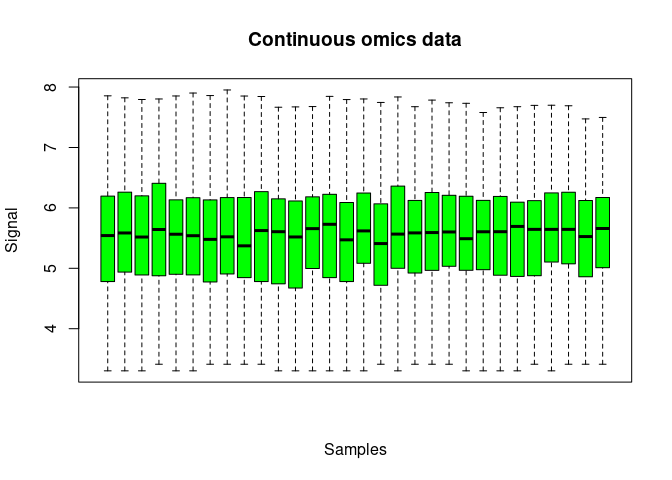We renamed metabolomicdata() to continuousomicdata() (while keeping the first name available) to offer its use to other continuous omic data such as proteomics data or RT-QPCR data. As for metabolomic data, the pretreatment of other continuous omic data data must be done before importation of data, and data must be imported in a scale that enables the use of a normal error model. This strong hypothesis is required both for selection of items and for dose-reponse modelling.

### 2.1.6 An example with continuous anchoring apical data

``anchoringfilename <- system.file("extdata", "apical_anchoring.txt", package = "DRomics")``

No transformation is provided in function continuousanchoringdata(). If needed the pretreatment of data must be done before importation of data, so that they can be directly modelled using a normal error model. This strong hypothesis is required both for selection of responsive endpoints and for dose-reponse modelling.

``(o.anchoring <- continuousanchoringdata(anchoringfilename, backgrounddose = 0.1))``
``````## Elements of the experimental design in order to check the coding of the data:
## Tested doses and number of replicates for each dose:
##
##    0  2.4  3.8  6.2 10.1 16.5 26.8 43.5 70.7
##   12    6    2    2    2    6    2    2    2
## Number of endpoints: 2
## Names of the endpoints:
##  "growth"         "photosynthesis"``````
``````# Here the argument backgrounddose is used to specify that doses below  or equal to 0.1
# are considered as 0 in the DRomics workflow.
# Specifying this argument is necessary when there is no doses at 0 in the data
plot(o.anchoring)``````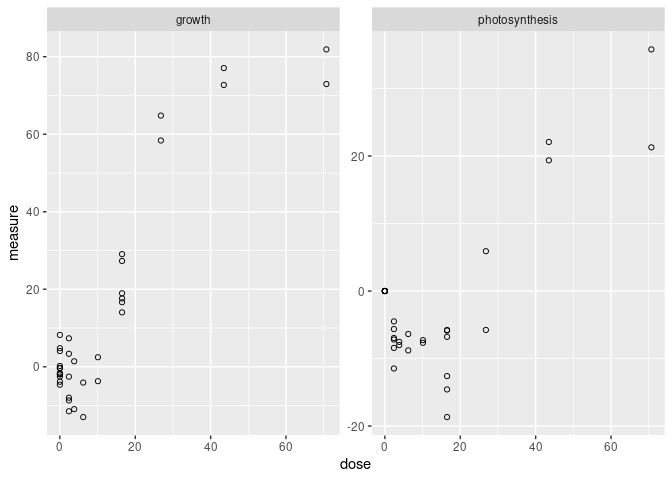For such data the plot() function provides a dose-response plot for each endpoint.

## 2.2 Step 2: selection of significantly responding items

For the second step of the workflow, function itemselect() must be used with the output of the function used in Step 1 as first argument (output of RNAseqdata(), microarraydata(), continuousomicdata() or continuousanchoringdata()). Below is an example with microarray data.

The false discovery rate (FDR) corresponds to the expected proportion of items that will be falsely detected as responsive. With a very large data set it is important to define a selection step based on an FDR not only to reduce the number of items to be further processed, but also to remove too noisy dose-response signals that may impair the quality of the results. We recommend to set a value between 0.001 and 0.1 depending of the initial number of items. When this number is very high (more than several tens of thousands), we recommend a FDR less than 0.05 (0.001 to 0.01) to increase the robustness of the results (Larras et al. 2018).

Concerning the method used for selection, we recommend the default choice (“quadratic”) for a typical omics dose-response design (many doses/concentrations with few replicates per condition). It enables the selection of both monotonic and biphasic dose-responses. If you want to focus on monotonic dose-responses, the “linear” method could be chosen. For a design with a small number of doses/concentrations and many replicates (not an optimal for dose-response modelling), the “ANOVA” method could be preferable.

See ?itemselect and Larras et al. 2018 for details.

``(s_quad <- itemselect(o.microarray, select.method = "quadratic", FDR = 0.01))``
``````## Number of selected items using a quadratic trend test with an FDR of 0.01: 150
## Identifiers of the first 20 most responsive items:
##   "383.2" "384.2" "363.1" "383.1" "384.1" "363.2" "364.2" "364.1" "300.2"
##  "301.1" "300.1" "301.2" "263.2" "27.2"  "25.1"  "368.1" "351.1" "15"
##  "370"   "350.2"``````

## 2.3 Step 3: fit of dose-response models, choice of the best fit for each curve

### 2.3.1 Fit

For Step 3 the function drcfit() must be simply used with the output of itemselect() as first argument. Description of the fitted models and of the procedure to select the best fit are described in Larras et al. 2018 and in ?drcfit. The former use of the AIC (Akaike criterion- default information criterion used for the selection of the best fit model in DRomics versions < 2.2-0) was replaced by the use of the AICc (second-order Akaike criterion) in order to prevent the overfitting that may occur with dose-response designs with a small number of data points, as recommended and now classically done in regression (Hurvich and Tsai, 1989; Burnham and Anderson DR, 2004).

As the call to this function may take time, by default a progressbar is provided. Some arguments of this function can be used to specify parallel computing to accelerate the computation (see ?drcfit for details).

``(f <- drcfit(s_quad, progressbar = FALSE))``
``````## Results of the fitting using the AICc to select the best fit model
## 22 dose-response curves out of 150 previously selected were removed
## because no model could be fitted reliably.
## Distribution of the chosen models among the 128 fitted dose-response curves:
##
##             Hill           linear      exponential     Gauss-probit
##                1               30               39               47
## log-Gauss-probit
##               11
## Distribution of the trends (curve shapes) among the 128 fitted dose-response curves:
##
##    U bell  dec  inc
##   19   38   37   34``````

In the following you can see the first ten lines of the output dataframe on our example (see ?drcfit for a complete description of the columns of the output dataframe.) This output dataframe provides information such as best-fit model, parameter value, coordinates of particular points, and the trend of the curve (among increasing, decreasing, U-shaped, bell-shaped)

``head(f\$fitres, 10)``
``````##       id irow adjpvalue        model nbpar       b    c     d     e    f SDres
## 1  383.2  725  2.08e-07 Gauss-probit     4  5.5836 8.58  8.58  1.70 3.62 0.157
## 2  384.2  727  2.08e-07  exponential     3 -0.0298   NA 12.24  1.76   NA 0.160
## 3  363.1  686  2.24e-07  exponential     3 -0.2058   NA  9.10  3.11   NA 0.218
## 4  383.1  724  2.24e-07 Gauss-probit     4  5.4879 8.75  8.75  1.72 3.58 0.169
## 5  384.1  726  3.41e-07 Gauss-probit     4  6.8453 7.26  7.26  1.77 5.01 0.158
## 6  363.2  687  7.01e-07  exponential     3 -0.1467   NA  9.10  2.77   NA 0.206
## 7  364.2  689  7.08e-07  exponential     3 -0.2289   NA  9.00  3.22   NA 0.249
## 8  364.1  688  8.37e-07  exponential     3 -0.1945   NA  9.06  3.02   NA 0.247
## 9  300.2  568  1.36e-06  exponential     3  2.4805   NA 11.15 -2.63   NA 0.522
## 10 301.1  569  1.36e-06  exponential     3  2.1243   NA 12.82 -1.71   NA 0.482
##         typology trend    y0 yrange xextrem yextrem
## 1        GP.bell  bell 12.04   1.17    1.70    12.2
## 2  E.dec.concave   dec 12.24   1.25      NA      NA
## 3  E.dec.concave   dec  9.10   1.53      NA      NA
## 4        GP.bell  bell 12.16   1.18    1.72    12.3
## 5        GP.bell  bell 12.10   1.11    1.77    12.3
## 6  E.dec.concave   dec  9.10   1.46      NA      NA
## 7  E.dec.concave   dec  9.00   1.56      NA      NA
## 8  E.dec.concave   dec  9.06   1.55      NA      NA
## 9   E.dec.convex   dec 11.15   2.28      NA      NA
## 10  E.dec.convex   dec 12.82   2.08      NA      NA``````

### 2.3.2 Plot of fitted curves

By default the plot() function used on the output of the drcfit() function provides the first 20 fitted curves (or the ones you specify using the argument items) with observed points. Fitted curves are represented in red, replicates are represented in open circles and means of replicates at each dose/concentration are represented by solid circles. All the fitted curves may be saved in a pdf file using the plotfit2pdf() function (see ?drcfit).

``plot(f) ``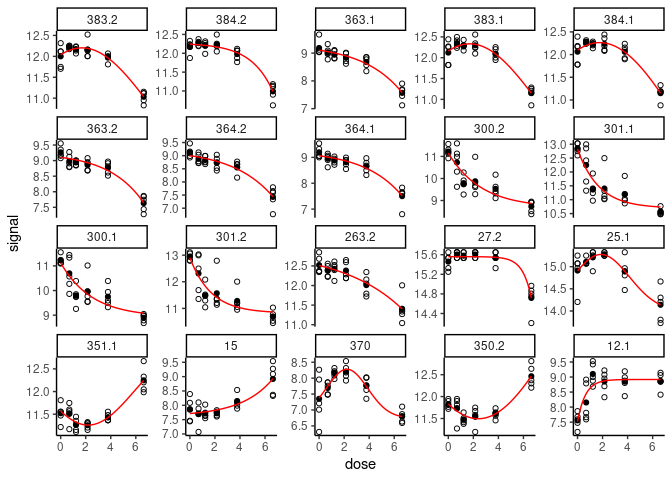The fitted curves may be represented using a log scale for the dose/concentration using argument dose_log_transfo (see ?drcfit for details and examples).

Another specific plot function named targetplot() can be used to plot targeted items, whether they were or not selected in step 2 and fitted in step 3. See an example below and details in ?targetplot

``````targetitems <- c("88.1", "1", "3", "15")
targetplot(targetitems, f = f)``````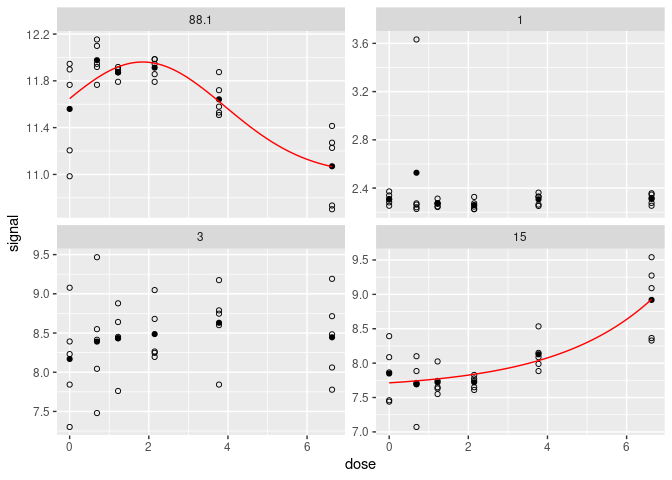### 2.3.3 Plot of residuals

To check the assumption of the normal error model, two types of residual plots can be used (“dose_residuals” or “fitted_residuals”). The residual plots for all items may also be saved in a pdf file using the plotfit2pdf() function (see ?drcfit).

``plot(f, plot.type = "dose_residuals")``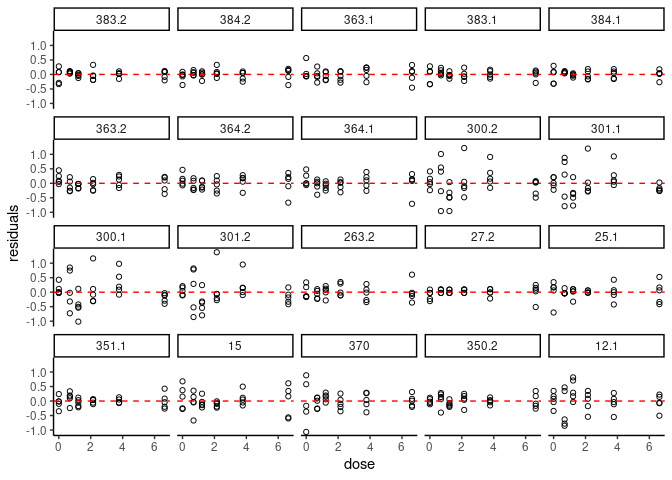## 2.4 Step 4: calculation of x-fold and z-SD benchmark doses

### 2.4.1 Calculation of BMD

The two types of benchmark doses (BMD-zSD and BMD-xfold) proposed by the EFSA (2017) are systematically calculated for each fitted dose-response curve using the function bmdcalc() with the output of the drcfit() function as a first argument (see Larras et al. 2018 or ?drcfit for details).

The argument z, by default at 1, is used to define the BMD-zSD as the dose at which the response is reaching y0 +/- z * SD, with y0 the level at the control given by the dose-response fitted model and SD the residual standard deviation of the dose-response fitted model.

The argument x, by default at 10 (for 10%), is used to define the BMD-xfold as the dose at which the response is reaching y0 +/- (x/100) * y0.

``(r <- bmdcalc(f, z = 1, x = 10))``
``````## 1 BMD-xfold values and 0 BMD-zSD values are not defined (coded NaN as
## the BMR stands outside the range of response values defined by the model).
## 61 BMD-xfold values and 0 BMD-zSD values could not be calculated (coded
## NA as the BMR stands within the range of response values defined by the
## model but outside the range of tested doses).``````

In the following you can see the first ten lines of the output dataframe of the function bmdcalc() on our example (see ?bmdcalc for a complete description of the columns of the output dataframe).

``head(r\$res, 10)``
``````##       id irow adjpvalue        model nbpar       b    c     d     e    f SDres
## 1  383.2  725  2.08e-07 Gauss-probit     4  5.5836 8.58  8.58  1.70 3.62 0.157
## 2  384.2  727  2.08e-07  exponential     3 -0.0298   NA 12.24  1.76   NA 0.160
## 3  363.1  686  2.24e-07  exponential     3 -0.2058   NA  9.10  3.11   NA 0.218
## 4  383.1  724  2.24e-07 Gauss-probit     4  5.4879 8.75  8.75  1.72 3.58 0.169
## 5  384.1  726  3.41e-07 Gauss-probit     4  6.8453 7.26  7.26  1.77 5.01 0.158
## 6  363.2  687  7.01e-07  exponential     3 -0.1467   NA  9.10  2.77   NA 0.206
## 7  364.2  689  7.08e-07  exponential     3 -0.2289   NA  9.00  3.22   NA 0.249
## 8  364.1  688  8.37e-07  exponential     3 -0.1945   NA  9.06  3.02   NA 0.247
## 9  300.2  568  1.36e-06  exponential     3  2.4805   NA 11.15 -2.63   NA 0.522
## 10 301.1  569  1.36e-06  exponential     3  2.1243   NA 12.82 -1.71   NA 0.482
##         typology trend    y0 yrange xextrem yextrem BMD.zSD BMR.zSD BMD.xfold
## 1        GP.bell  bell 12.04   1.17    1.70    12.2    1.33   12.19        NA
## 2  E.dec.concave   dec 12.24   1.25      NA      NA    3.26   12.08      6.59
## 3  E.dec.concave   dec  9.10   1.53      NA      NA    2.25    8.89      5.26
## 4        GP.bell  bell 12.16   1.18    1.72    12.3    1.52   12.33        NA
## 5        GP.bell  bell 12.10   1.11    1.77    12.3    1.41   12.26        NA
## 6  E.dec.concave   dec  9.10   1.46      NA      NA    2.43    8.90      5.47
## 7  E.dec.concave   dec  9.00   1.56      NA      NA    2.38    8.75      5.14
## 8  E.dec.concave   dec  9.06   1.55      NA      NA    2.47    8.81      5.24
## 9   E.dec.convex   dec 11.15   2.28      NA      NA    0.62   10.63      1.57
## 10  E.dec.convex   dec 12.82   2.08      NA      NA    0.44   12.34      1.58
##    BMR.xfold
## 1      10.83
## 2      11.02
## 3       8.19
## 4      10.95
## 5      10.89
## 6       8.19
## 7       8.10
## 8       8.15
## 9      10.04
## 10     11.54``````

### 2.4.2 Various plots of the BMD distribution

The default plot of the output of the bmdcalc() function provides the distribution of benchmark doses as an ECDF (Empirical Cumulative Density Function) plot for the chosen BMD (“zSD”" or “xfold”). See an example below.

``plot(r, BMDtype = "zSD", plottype = "ecdf") ``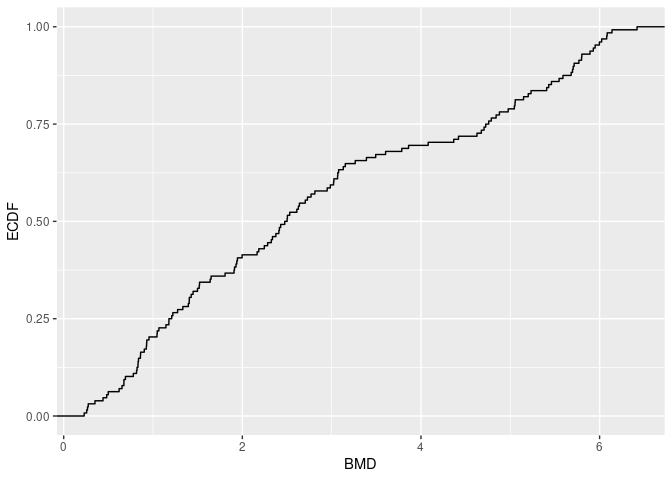Different alternative plots are proposed (see ?bmdcalc for details) that can be obtained using the argument plottype to choose the type of plot (“ecdf”, “hist” or “density”) and the argument by to split the plot by “trend”, “model” or “typology”. Below is an example of a density plot of BMD-zSD split by trend of dose-response curves.

``plot(r, BMDtype = "zSD", plottype = "density", by = "trend") ``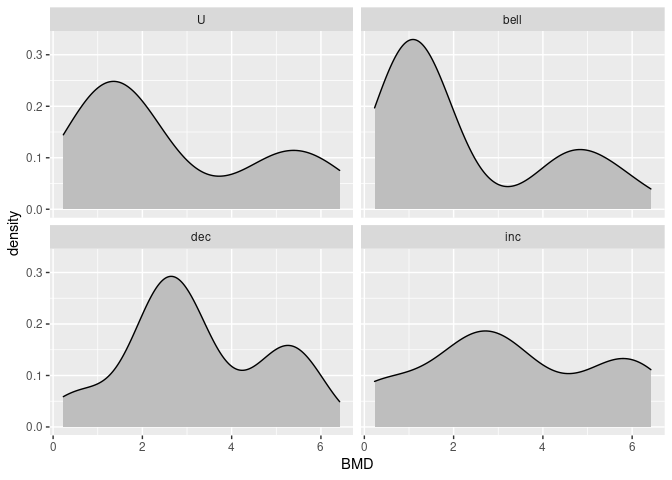### 2.4.3 Plot of BMD distribution with a color gradient for signal intensity

On a BMD ECDF plot one can add of a color gradient for each item coding for the intensity of the signal (after shift of the control signal at 0) as a function of the dose (see ?bmdplotwithgradient for details and an example below).

``````bmdplotwithgradient(r\$res, BMDtype = "zSD",
facetby = "trend",
shapeby = "model",
line.size = 1.2) + labs(shape = "model")``````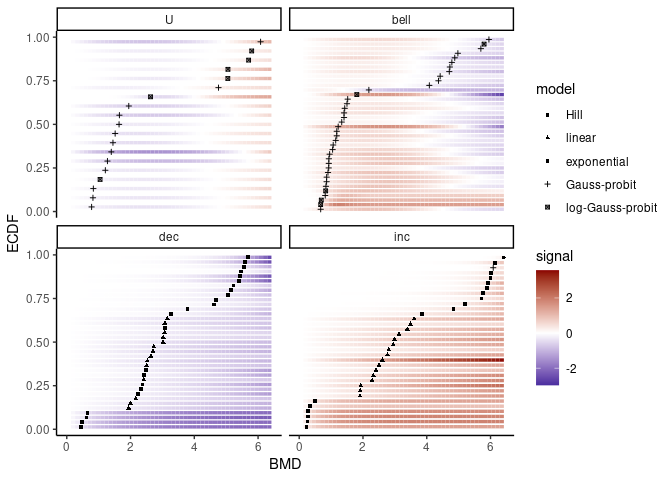As in the previous example, you can use the argument line.size to manually adjust the width of lines in that plot if the default value does not give a visual result that suits you.

### 2.4.4 Plot of fitted curves with BMD values

It is possible to add the output of bmdcalc() in the argument BMDoutput of the plot() function of drcfit() to add BMD values (when defined) as a vertical line on each fitted curve. Horizontal dotted lines corresponding to the two BMR potential values will be also added. All the fitted curves may also be saved in the same way in a pdf file using the plotfit2pdf() function (see ?drcfit).

``plot(f, BMDoutput = r) ``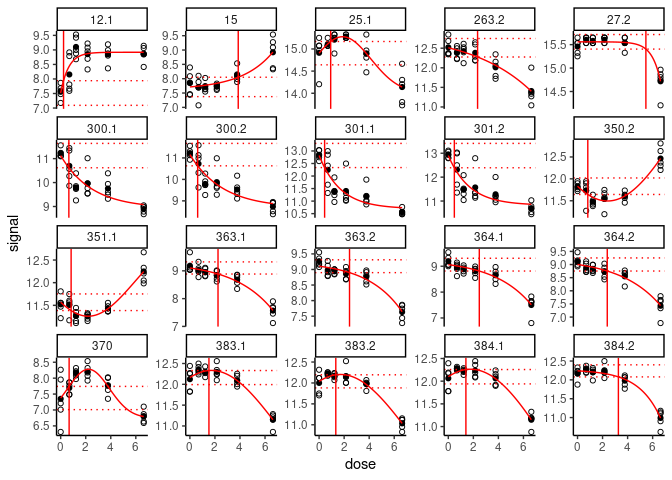## 2.5 Step 5: calculation of confidence intervals on the BMDs by bootstrap

Confidence intervals on BMD values can be calculated by bootstrap. As the call to this function may take much time, by default a progressbar is provided and some arguments can be used to specify parallel computing to accelerate the computation (see ?bmdboot for details).

In the example below a small number of iterations was used just to make this vignette quick to compile, but the default value of the argument niter (1000) should be considered as a minimal value to obtain stable results.

### 2.5.1 Bootstrap calculation

``(b <- bmdboot(r, niter = 50, progressbar = FALSE))``
``````## Bootstrap confidence interval computation failed on 16 items among 128
## due to lack of convergence of the model fit for a fraction of the
## bootstrapped samples greater than 0.5.
## For 6 BMD.zSD values and 69 BMD.xfold values among 128 at least one
## bound of the 95 percent confidence interval could not be computed due
## to some bootstrapped BMD values not reachable due to model asymptotes
## or reached outside the range of tested doses (bounds coded Inf)).``````

This function gives an output corresponding to the output of the bmdcalc() function completed with bounds of BMD confidence intervals (by default 95% confidence intervals).

``head(b\$res, 10)``
``````##       id irow adjpvalue        model nbpar       b    c     d     e    f SDres
## 1  383.2  725  2.08e-07 Gauss-probit     4  5.5836 8.58  8.58  1.70 3.62 0.157
## 2  384.2  727  2.08e-07  exponential     3 -0.0298   NA 12.24  1.76   NA 0.160
## 3  363.1  686  2.24e-07  exponential     3 -0.2058   NA  9.10  3.11   NA 0.218
## 4  383.1  724  2.24e-07 Gauss-probit     4  5.4879 8.75  8.75  1.72 3.58 0.169
## 5  384.1  726  3.41e-07 Gauss-probit     4  6.8453 7.26  7.26  1.77 5.01 0.158
## 6  363.2  687  7.01e-07  exponential     3 -0.1467   NA  9.10  2.77   NA 0.206
## 7  364.2  689  7.08e-07  exponential     3 -0.2289   NA  9.00  3.22   NA 0.249
## 8  364.1  688  8.37e-07  exponential     3 -0.1945   NA  9.06  3.02   NA 0.247
## 9  300.2  568  1.36e-06  exponential     3  2.4805   NA 11.15 -2.63   NA 0.522
## 10 301.1  569  1.36e-06  exponential     3  2.1243   NA 12.82 -1.71   NA 0.482
##         typology trend    y0 yrange xextrem yextrem BMD.zSD BMR.zSD BMD.xfold
## 1        GP.bell  bell 12.04   1.17    1.70    12.2    1.33   12.19        NA
## 2  E.dec.concave   dec 12.24   1.25      NA      NA    3.26   12.08      6.59
## 3  E.dec.concave   dec  9.10   1.53      NA      NA    2.25    8.89      5.26
## 4        GP.bell  bell 12.16   1.18    1.72    12.3    1.52   12.33        NA
## 5        GP.bell  bell 12.10   1.11    1.77    12.3    1.41   12.26        NA
## 6  E.dec.concave   dec  9.10   1.46      NA      NA    2.43    8.90      5.47
## 7  E.dec.concave   dec  9.00   1.56      NA      NA    2.38    8.75      5.14
## 8  E.dec.concave   dec  9.06   1.55      NA      NA    2.47    8.81      5.24
## 9   E.dec.convex   dec 11.15   2.28      NA      NA    0.62   10.63      1.57
## 10  E.dec.convex   dec 12.82   2.08      NA      NA    0.44   12.34      1.58
##    BMR.xfold BMD.zSD.lower BMD.zSD.upper BMD.xfold.lower BMD.xfold.upper
## 1      10.83         0.489         3.934             Inf             Inf
## 2      11.02         1.724         4.578           6.400             Inf
## 3       8.19         1.366         3.519           4.606            5.96
## 4      10.95         0.423         4.169             Inf             Inf
## 5      10.89         0.480         4.270             Inf             Inf
## 6       8.19         1.334         3.306           4.751            6.00
## 7       8.10         1.062         3.245           4.247            5.49
## 8       8.15         1.363         3.535           4.114            5.77
## 9      10.04         0.308         0.857           1.020            2.02
## 10     11.54         0.226         0.689           0.936            2.42
##    nboot.successful
## 1                36
## 2                47
## 3                50
## 4                36
## 5                25
## 6                50
## 7                50
## 8                50
## 9                50
## 10               50``````

### 2.5.2 Add of confidence intervals on BMD ECDF plots

The plot() function applied on the output the bmdboot() function gives an ECDF plot of the chosen BMD with the confidence interval of each BMD (see an example below). By default BMDs with an infinite confidence interval bound are not plotted.

``plot(b, BMDtype = "zSD", by = "trend") ``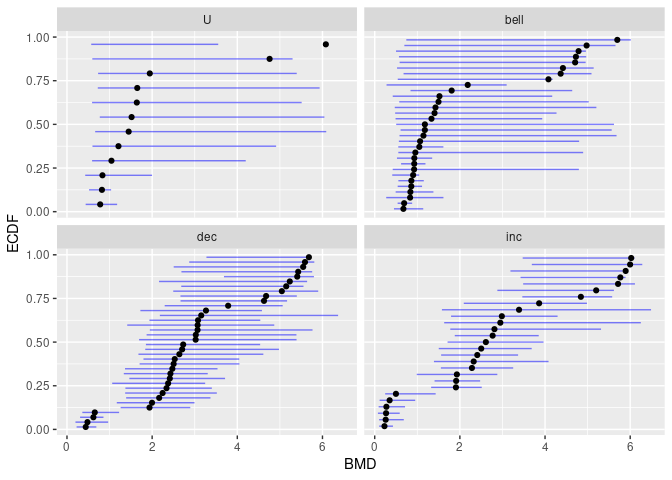### 2.5.3 Plot of fitted curves with BMD values and confidence intervals

It is possible to add the output of bmdboot() in the argument BMDoutput of the plot() function of drcfit() to add BMD values (when defined) as a vertical line on each fitted curve and bounds of the confidence intervals (when successfully calculated) as two dashed lines. Horizontal dotted lines corresponding to the two BMR potential values will be also added. All the fitted curves may also be saved in the same way in a pdf file using the plotfit2pdf() function (see ?drcfit).

``plot(f, BMDoutput = b) ``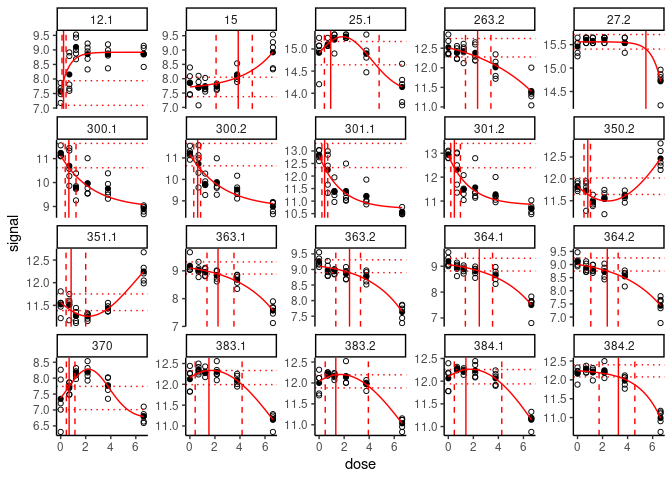# 3 Help for biological interpretation of DRomics outputs

This section illustrates functions of DRomics that are meant to help the biological interpretation of outputs. The idea is to augment the output dataframe with new column(s) bringing biological information such as provided by functional annotation of the items (e.g. KEGG pathway classes or GO terms) then to use this information to organize the visualisation of the DRomics output.

Below is used an example from a metabolomic data set previously analysed using DRomics.

## 3.1 Enrichment of the dataframe of DRomics results with functional annotation

This enrichment is not done using DRomics functions, but with relevant R functions such as merge().

An example of how to proceed:

1. Import the dataframe with DRomics results to be used: the output \$res of bmdcalc() or bmdboot() functions from step 4 or 5 of the main DRomics workflow.

(This step will not be necessary if previous steps are done directly in R using the DRomics package as described previously in this vignette. We did it to take a real example that took a long time to run but from which results are stored in the package.)

``````# code to import the file for this example in our package
resfilename <- system.file("extdata", "triclosanSVmetabres.txt", package = "DRomics")

# to see the structure of this file
str(res)``````
``````## 'data.frame':    31 obs. of  14 variables:
##  \$ id           : Factor w/ 31 levels "NAP47_51","NAP_2",..: 2 3 4 5 6 7 8 9 10 11 ...
##  \$ model        : Factor w/ 4 levels "Gauss-probit",..: 2 2 3 2 2 4 2 2 3 3 ...
##  \$ y0           : num  5.94 5.36 7.86 6.86 6.21 ...
##  \$ b            : num  0.4598 -0.1976 -0.0451 0.6011 0.6721 ...
##  \$ c            : num  NA NA NA NA NA ...
##  \$ d            : num  5.94 5.36 7.86 6.86 6.21 ...
##  \$ e            : num  -1.648 6.323 NA -0.321 -0.323 ...
##  \$ f            : num  NA NA NA NA NA ...
##  \$ yrange       : num  0.456 0.477 0.35 0.601 0.672 ...
##  \$ trend        : Factor w/ 4 levels "U","bell","dec",..: 3 3 3 3 3 1 3 3 3 3 ...
##  \$ typology     : Factor w/ 10 levels "E.dec.concave",..: 2 1 7 2 2 9 2 2 7 7 ...
##  \$ BMD.zSD      : num  0.528 2.075 1.154 0.158 0.182 ...
##  \$ BMD.zSD.lower: num  0.2176 1.0519 0.7722 0.0542 0.0694 ...
##  \$ BMD.zSD.upper: num  1.074 3.375 1.496 0.584 0.74 ...``````
1. Import the dataframe with functional annotation (or any other descriptor/category you want to use, here KEGG pathway classes) of each item present in the ‘res’ file.

Examples are embedded in the DRomics package, but be cautious, generally this file must be produced by the user. Each item may have more than one annotation (i.e. more than one line).

``````# code to import the file for this example in our package
annotfilename <- system.file("extdata", "triclosanSVmetabannot.txt", package = "DRomics")

# to see the structure of this file
str(annot)``````
``````## 'data.frame':    84 obs. of  2 variables:
##  \$ metab.code: Factor w/ 31 levels "NAP47_51","NAP_2",..: 2 3 4 4 4 4 5 6 7 8 ...
##  \$ path_class: Factor w/ 9 levels "Amino acid metabolism",..: 5 3 3 2 6 8 5 5 5 5 ...``````
1. Merging of both previous dataframes in order to obtain a so-called ‘extendedres’ dataframe gathering, for each item, metrics derived from the DRomics workflow and functional annotation.

Arguments by.x and by.y of the merge() function indicate the column name in res and annot dataframes, respectively, that must be used for the merging.

``````annotres <- merge(x = res, y = annot, by.x = "id", by.y = "metab.code")
``````##         id       model   y0       b  c    d     e  f yrange trend      typology
## 1 NAP47_51      linear 7.34 -0.0560 NA 7.34    NA NA  0.435   dec         L.dec
## 2    NAP_2 exponential 5.94  0.4598 NA 5.94 -1.65 NA  0.456   dec  E.dec.convex
## 3   NAP_23 exponential 5.36 -0.1976 NA 5.36  6.32 NA  0.477   dec E.dec.concave
## 4   NAP_30      linear 7.86 -0.0451 NA 7.86    NA NA  0.350   dec         L.dec
## 5   NAP_30      linear 7.86 -0.0451 NA 7.86    NA NA  0.350   dec         L.dec
## 6   NAP_30      linear 7.86 -0.0451 NA 7.86    NA NA  0.350   dec         L.dec
##   BMD.zSD BMD.zSD.lower BMD.zSD.upper
## 1   2.224         0.991          4.22
## 2   0.528         0.218          1.07
## 3   2.075         1.052          3.38
## 4   1.154         0.772          1.50
## 5   1.154         0.772          1.50
## 6   1.154         0.772          1.50
##                                    path_class
## 1                            Lipid metabolism
## 2                            Lipid metabolism
## 3                     Carbohydrate metabolism
## 4                     Carbohydrate metabolism
## 5 Biosynthesis of other secondary metabolites
## 6                          Membrane transport``````

## 3.2 BMD ECDF plot by functional group

### 3.2.1 BMD ECDF plot split by group defined from functional annotation

Using the function bmdplot() and its argument facetby, the BMD ECDF plot can be split by group (here by KEGG pathway class). Confidence intervals can be added on this plot and color coding for trend in this example (See ?bmdplot for more options).

``````bmdplot(annotres, BMDtype = "zSD", add.CI = TRUE,
facetby = "path_class",
colorby = "trend") + labs(col = "trend")``````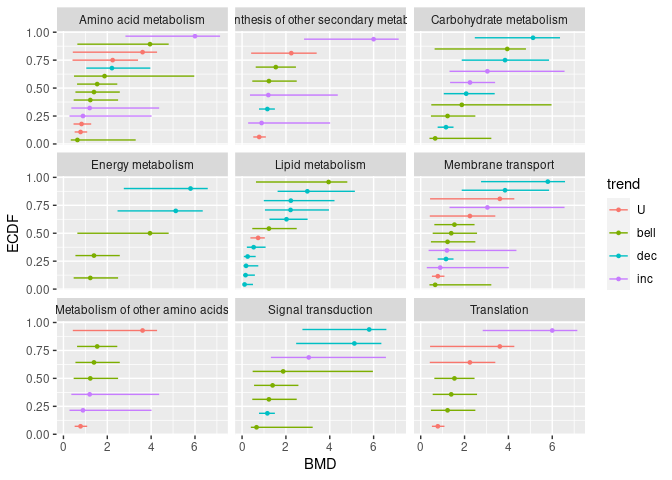Function ecdfplotwithCI() can also used as an alternative as below to provide the same plot differing just by the coloring of intervals only. (See ?ecdfplotwithCI for more options.)

``````ecdfplotwithCI(variable = annotres\$BMD.zSD,
CI.lower = annotres\$BMD.zSD.lower,
CI.upper = annotres\$BMD.zSD.upper,
by = annotres\$path_class,
CI.col = annotres\$trend) + labs(col = "trend")``````

### 3.2.2 BMD ECDF plot with color gradient split by group defined from functional annotation

Using the function bmdplotwithgradient() and its argument facetby, the BMD plot with color gradient can be split here by KEGG pathway class. (See ?bmdplotwithgradient for more options).

``````bmdplotwithgradient(annotres, BMDtype = "zSD",
facetby = "path_class",
shapeby = "trend") + labs(shape = "trend")``````